# 07 朴素叶贝斯算法

## 概率基础

1. 概率： 一件事情发生的可能性
2. 联合概率： 包含多个条件，且所有条件同时成立的概率。P(A,B)
P(A, B) = P(A)P(B)
3. 条件概率：事件A在另外一个事件B已经发生条件下发生的概率。 P(A|B)
P(A1,A2 | B) = P(A1 | B) * P(A2 | B)
注意： 此条件概率的成立，是由于A1, A2相互独立的结果

## 朴素贝叶斯

1. 朴素： 特征独立，常用文档分类
• 在给定?#26102;?#20363;的基础上，求各类型文档的比例
2. 贝叶斯公式： （多个条件下一个结果）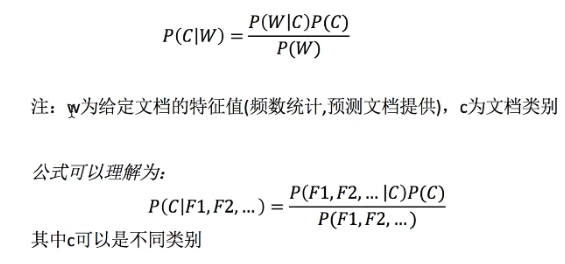• 公式分为3个部分：
• P(C): 每个文档类别的概率 （某类文档数/总文档数）
• P(W | C)：给定类别下特征（被预测文档中出现的词）的概率：计算方法：P(F1|C） = Ni/N
• Ni : F1词在C类别文档所有文档出现的次数
• N: 所属C类别下的文档所有词出现的次数和
• P(F1,F2,F3) : 预测文档中每个词的概率
1. 文档分类： 给定一个文档的条件下，求文档所属于科技、娱乐等类别的概率。哪个类别的概率大，则归为某个类别。
• 文档：词1， 词2 ， 词3 （词出现的数量的情况下，判断类别）
• P(科技|词1，词2，词3） = P(f1,f2,f3 | 科技）*P(科技）/P(W)
• P(娱乐|词1，词2，词3） = P(f1,f2,f3 | 娱乐）*P(娱乐）/P(W)
• 由于是概率大小，则P(W)可以同时约去

### 文档分类实例

• 科技： P(科技 |影院，支付宝，云计算）= P(影院，支付宝，云计算| 科技）* P(科技）= P(影院|科技）P(支付宝|科技） P(云计算|科技）P(科技）= （8/100）* （20/100）* （63/100）*（30/90） = 0.00456109
• 娱乐：P(娱乐 |影院，支付宝，云计算）= （56/121）（15/121）（0/121）（60/90）= 0

### 拉普拉斯平滑

1. 目的：避免单个特征词出现次数为0 ，导致最终的计算结果为0 。
2. 原因：其他的词在这个类型文档中出现过，则还是有可能属于这个文档。
3. P(F1|C) = (Ni +a) /(N+am)
• a为指定的系数，一般取1
• m为训练文档?#22411;?#35745;出的特征词的个数 （上述例子中为4）

### sklearn朴素贝叶斯API

sklearn.naive_bayes.MultinomialNB (alpha = 1.0)

### 朴素贝叶斯算法案例 （sklearn 20类新闻分类）

#### 案例流程

1. 加载数据，进行分割
2. 生成文章特征词
3. 朴素贝叶斯estimator进?#24615;?#20272;

### 算法总结

1. 训练集误差大，结果肯定不好
2. 不需要调参
3. 优点：
• 发源于古典数学理论，有稳定的分类效率
• 对?#31508;?#25968;据不太敏感，算法也比较简单，常用于文本分类
• 分类准确率高，速度快
4. 缺点：
• 由于使用样本独立性假设，对样本属性有关联时效果不好

## 分类模型评估

### 常见评估方法

1. estimator.score() - 准确率，预测结果正确的百分比
2. 精确率（precision) - 预测结果为正例样本中真实为正例的比例（查的准）
3. 召回率（recall) - 真实为正例的样本?#24615;?#27979;结果为正例的比例（查的全）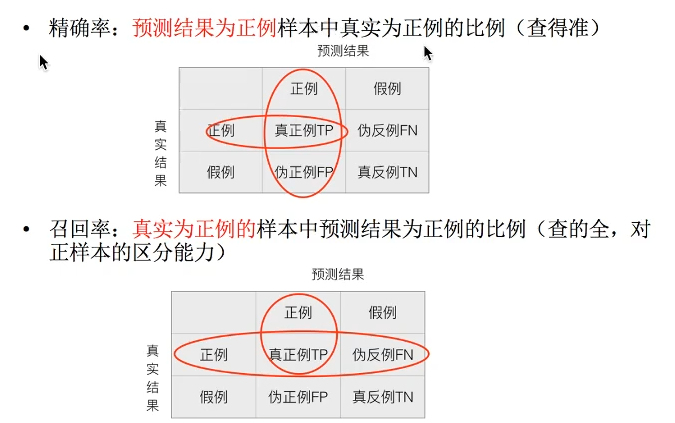4. F1-score - 反映了模型的稳定性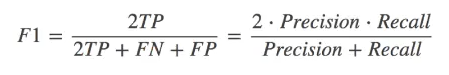### 混淆矩阵

1. 定义：在分类任务中，预测结果（Predicted condition) 与正确标记 （True condition)之间存在四种不同的组合，构成混淆矩阵。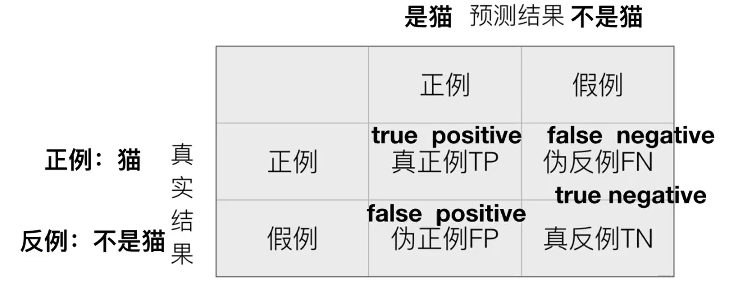### 分类评估API

sklearn.metrics.classification_report(y_true, y_pred, target_names=None)

• y_true: 真?#30340;?#26631;值
• y_pred: 估计器预测目标值
• target_names: 目标类别名称
• return: 每个类别精确率和召回率

### 案例代码

from sklearn.datasets import fetch_20newsgroups
from sklearn.feature_extraction.text import TfidfVectorizer
from sklearn.model_selection import train_test_split
from sklearn.naive_bayes import MultinomialNB
from sklearn.metrics import classification_report

def naivebayes():
"""
朴素贝叶斯进行文本分类
:return: None
"""
news = fetch_20newsgroups(subset='all')
# 进行数据分割
x_train, x_test, y_train, y_test = train_test_split(news.data, news.target_names, test_size=0.25 )

# 对数据集进行特征抽取
tf = TfidfVectorizer()

# 以训练集当中的词的列表进行每篇文章重要性统计
x_train = tf.fit_transform(x_train)
print(tf.get_feature_names())
x_test = tf.transform(x_test)

# 进行朴素贝叶斯算法的计算
mlt = MultinomialNB(alpha=1.0)
mlt.fit(x_train, y_train)
print(x_train)
y_predict = mlt.predict(x_test)

print("预测的文章类别为：", y_predict)
score = mlt.score(x_test, y_test)
print("分类准确率为：", score)

print("每个类别的精确率和召回率：", classification_report
(y_test,y_predict,target_names=news.target_names))
return None

if __name__ == '__main__':
naivebayes()

## 模型的选择与调优

### 交叉验证过程

1. 定义： 将拿到的训练数据，氛围训练和验证集。将数据分为5份，其中一份作为验证集，然后经过5次（组）的测试，每次都更换不同的验证集。即得到5组模型的结果，取平均值作为最终结果。又称为5折交叉验证。

2. 图示：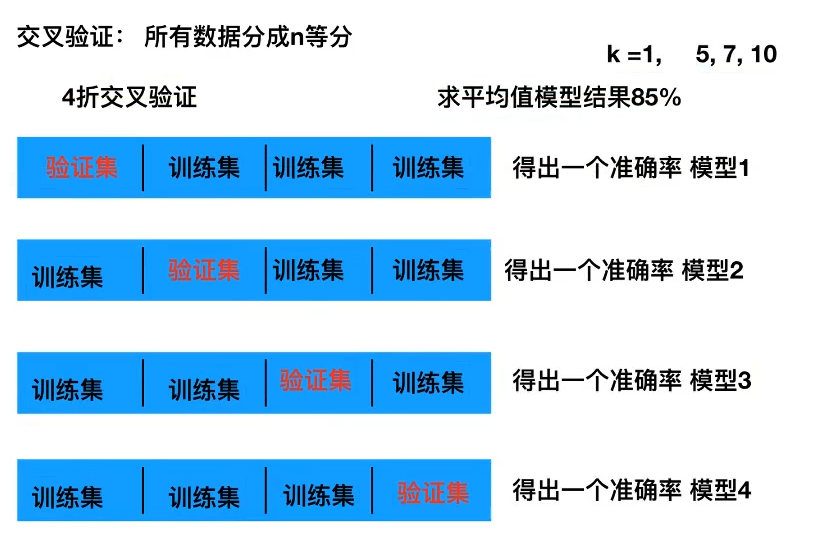### 超参数搜索 - 网格搜索

1. 超参数?#21644;?#24120;情况下，有很多的参数是需要手动指定的（如K-近邻算法中的K值），这种参数就是超参数。 （外部可以调整的参数）

2. 网格搜索：手动的调参过程繁杂，所以需要?#38405;?#22411;预设集中超参数组合，每组超参数都采用交叉验证来进行评估，最后选择最优参数组合建立模型。

3. 多个参数时：需要进行两两组合，或者多多组合。

### sklearn API

• sklearn.model_selection.GridSearchCV(estimator,param_grid=None,cv=None) (CV: cross validation)

• 对估计器的指定参数值进行详尽搜索
• estimator: 估计器对象
• param_grid: 估计器参数（dict): {"n_neighbors": [1,3,5]}
• cv: 指定几折交叉验证
• fit: 输入训练数据
• score: 准确率
• best_score_: 在交叉验证中验证的最好结果
• best_estimator_: 最好的参数模型
• cv_results_: 每次交叉验证后的测?#32422;?#20934;确率结果和训练集准确率结果
from sklearn.neighbors import KNeighborsClassifier
from sklearn.preprocessing import StandardScaler
from sklearn.model_selection import train_test_split,GridSearchCV
import pandas as pd

def knncls():
"""
K-近邻预测用户签到位置
:return: None
"""
# 读取数据

# 处理数据
# 1.缩小数据
data = data.query('x >1.0 & x < 1.25 & y > 2.5 & y < 2.75')

# 2. 处理时间数据
time_value = pd.to_datetime(data['time'], unit='s')
# print(time_value)

# 3. 构造一些特征, 把日期格式转换为字典格式
time_value = pd.DatetimeIndex(time_value)
data['day'] = time_value.day
data['weekday'] = time_value.weekday
data['hour'] = time_value.hour

# 4.把时间戳特征删除
data = data.drop(['time'],axis=1)
# print(data)

# 5.把签到数?#21487;?#20110;n个的目标位置删除
place_count = data.groupby('place_id').count() # 统计place_id次数,变成行索引
tf = place_count[place_count.row_id > 3].reset_index() # row_id 具体次数，reset_index 把place_id生成新的一列
data = data[data['place_id'].isin(tf.place_id)] # 筛选place_id
# print(data)

# 6.取出数据中的特征值和目标值
y = data['place_id']
x = data.drop(['place_id', 'row_id'], axis=1) # 去掉row_id 可以增加精度

# 7.分割训练集和测?#32422;?    x_train, x_test, y_train, y_text = train_test_split(x, y, test_size=0.25)

# 8.特征工程（标准化）
std = StandardScaler()
x_train = std.fit_transform(x_train)
x_test = std.transform(x_test)

# 进行算法流程 fit, predict, score  #超参数
knn = KNeighborsClassifier() # 使用网格搜索时，不用设定超参数
# knn.fit(x_train, y_train)
#
# # 得出预测结果
# y_predict = knn.predict(x_test)
# print('预测的目标签到位置为：', y_predict)
#
# # 得出准确率
# print('预测的准确率为：', knn.score(x_test, y_text))

# 9. 进?#22411;?#26684;搜索
param = {'n_neighbors': [3,5,10]}
gc = GridSearchCV(knn, param_grid=param, cv=2)
gc.fit(x_train, y_train)

# 10.预测准确率
print("在测?#32422;?#19978;的准确率：", gc.score(x_test, y_text))
print("在交叉验证中最好的结果：", gc.best_score_)
print('选择的最好的模型：', gc.best_estimator_)
print('每个超参数每次验证的结果：', gc.cv_results_)

return None

if __name__ == '__main__':
knncls()
posted @ 2019-11-21 23:20  hp_lake  阅读(...)  评论(... 编辑 收藏